Courses

# Motion - Physics, IAS UPSC Notes | EduRev

## UPSC : Motion - Physics, IAS UPSC Notes | EduRev

The document Motion - Physics, IAS UPSC Notes | EduRev is a part of the UPSC Course General Science(Prelims) by IRS Divey Sethi.
All you need of UPSC at this link: UPSC

Motion

• Speed is the rate of change of distance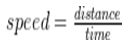• Velocity is the rate of change of displacement. It signifies both the speed and the direction of movement of an object.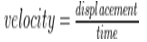• DISTANCE–TIME GRAPHS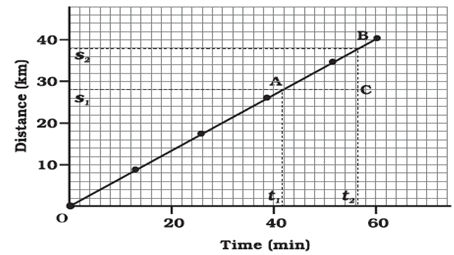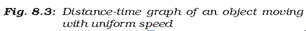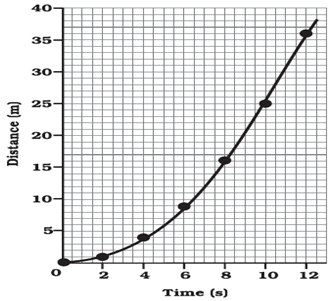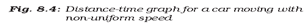• Acceleration is the rate of change of velocity.• Acceleration due to gravity is the acceleration experienced by an object as it falls freely towards the ground. It is constant throughout the surface of the earth.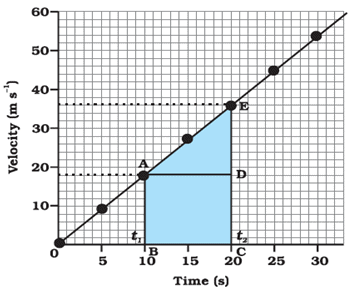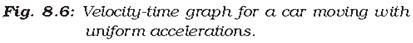VELOCITY-TIME GRAPHS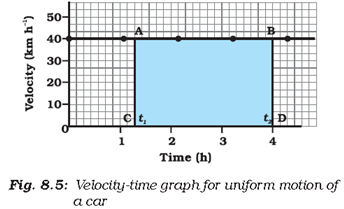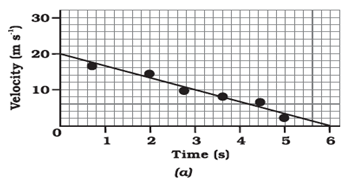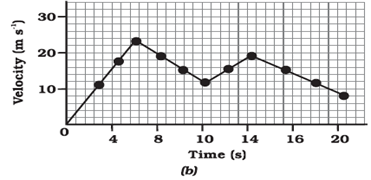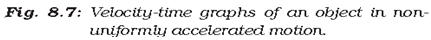Circular motion

• When a particle moves with a constant speed in a horizontal circular path about an axis or a definite point assumed to be a centre, its motion is called the uniform circular motion.

Centripetal force

• when a particle or a body moves with a uniform speed v on a circular path of radius r, then it is acted upon by a force mv2/r which is always directed towards the centre of the circle. This force is called centripetal force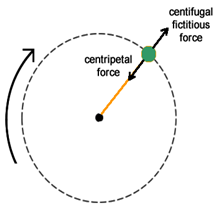Newton’s laws of motion

• First law:  Every body continues to be in its state of rest or of uniform motion in a straight line unless compelled by some external force to act otherwise. The first law of motion is related to the law of inertia

Inertia

• Inertia is a tendency of all objects and matter in the universe to remain still, or, if moving, to continue moving in the same direction unless acted on by some outside force.

Inertia of body is of three types:

• Inertia at rest: It is the inability of body to change by itself, its state of rest. This means a body at rest will remain at rest and cannot start moving on its own.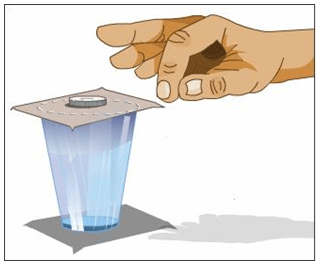• Inertia of motion: It is the inability of a body to change by itself, its state of uniform motion can neither accelerate nor retards on its own and comes to rest.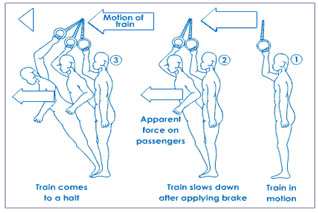• Inertia of direction: It is the inability of the body to change by itself its direction of motion, i.e., a body continues to move along the same straight line unless compelled by some external force to change it.

Newton's First Law Example

1) Why do the dry leaves and fruits fall when we shake the tree?
When we shake the tree the fruits and dry leaves remain at their position due to first law of motion, the inertia resist the motion, so, as the branches shake, they get detached from the tree.
2) Passengers fall forward when bus suddenly stops. Why?
The passengers are in motion due to first law and as the bus suddenly stops due to inertia their upper body opposes the force and continues to remain in motion.

Second Law of Motion

• Newton's second law of motion states that rate of change of momentum is directly proportional to applied force and takes place in the same direction as the applied force.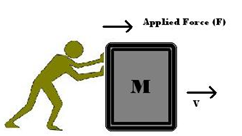• Momentum,
Momentum is the product of mass of the body and its velocity. p = mass x velocity
• What is Impulse?
Impulse can be defined as the force per unit time or change in momentum.
• Momentum is changed whenever a force is applied to a body.
•         Impulse = Force x Time

= Change in momentum

What is Impulse?

• Impulse can be defined as the force per unit time or change in momentum.
• Momentum is changed whenever a force is applied to a body.
•         Impulse = Force x Time

= Change in momentum

Newton's Second Law Example

1) In case of an apple falling from a tree, the apple is accelerated. So, its velocity changes as it is hanging on the tree and moves towards the ground.
Thus, by Newton's 2nd law there must be a force that acts on the apple to cause this acceleration. If we call this force "gravity", then the associated acceleration is "acceleration due to gravity".

To every action there is equal and opposite reaction

1.)In case of inflated balloon, the air rushing outward is action while the balloon going upward is reaction

2) Same thing happens in the rocket propulsion.
The rocket leaves or exerts fuel with some force, the fuel in return produces an equal force on the rocket, which makes the rocket accelerate in the forward direction.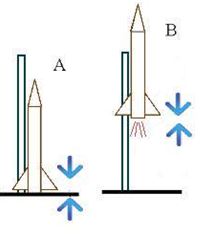COMMON FORCES IN MECHANICS

Friction

• Friction is the force resisting the relative motion of solid surfaces, fluid layers, and material elements sliding against each other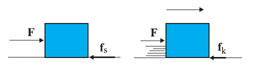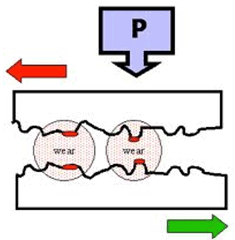Offer running on EduRev: Apply code STAYHOME200 to get INR 200 off on our premium plan EduRev Infinity!

## General Science(Prelims) by IRS Divey Sethi

39 videos|110 docs|255 tests

,

,

,

,

,

,

,

,

,

,

,

,

,

,

,

,

,

,

,

,

,

,

,

,

;